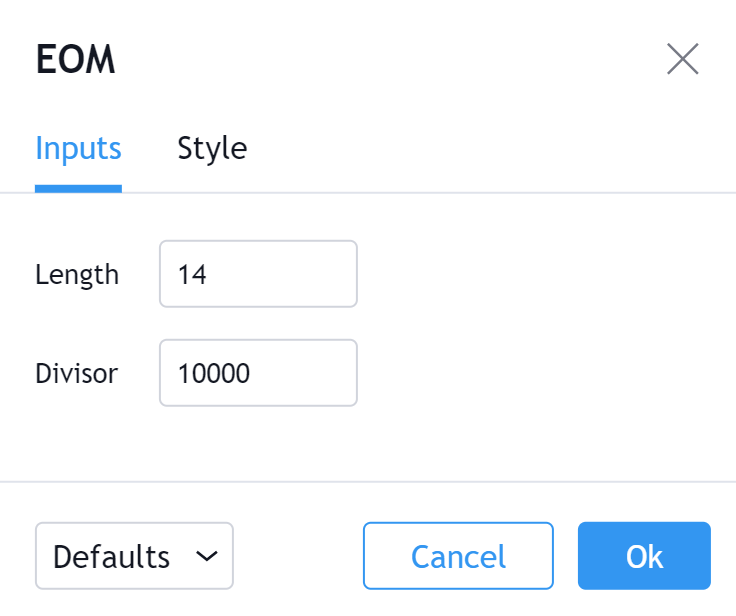# Ease of Movement (EOM)

#### Definition

The Ease of Movement indicator is a volume based oscillator. It is designed to measure the relationship between price and volume and display that relationship as an oscillator that fluctuates between positive and negative values. The EOM fluctuates above and below a Zero Line. This is done in order to quantify the "ease" of price movements. A basic understanding is that when the EOM is in positive territory, prices are advancing with relative ease. When the EOM is negative, prices are declining with relative ease.

#### History

The Ease of Movement indicator (EOM) was developed and introduced by Richard Arms.

#### Calculation

There are four necessary steps when calculating Ease of Movement for this example we will calculate a 14 Period EOM which is very common:
1. Calculate the distance moved.

`((CurrentHigh + CurrentLow)/2 - (PreviousHigh + PreviousLow)/2) = Distance Moved`

2. Calculate the Box Ratio which take volume and high/low range to produce the denominator in EOM calculations.

`((CurrentVolume/100,000,000) / (CurrentHigh - CurrentLow))`

3. Calculate a 1 Period EOM.

`Distance Moved / Box Ratio = 1 Period EOM`

4. Calculate a 14 Period EOM.

`14 Period Simple Moving Average of the 1 Period EOM = 14 Period EOM`

#### The basics

As previously mentioned, EOM plots a line which fluctuates between positive and negative values. Simply put, when the values are positive, price is advancing. When numbers are negative, prices are declining. One has to remember from the above calculations that price distance moved determines whether the EOM line is positive or negative. The Box Ratio, (which is determined by (volume)) is what determines the strength or range of that move. Therefore, when it comes to analyzing EOM, keep two points in mind.
A wide range with low volume indicates a high level of ease of movement.
A narrow range with high volume indicates a low level of ease of movement.

Because of the role that volume plays in the Ease of Movement indicator, many technical analysts use a standard Volume indicator in conjunction with the EOM.

#### What to look for

##### Trend Direction

Identifying basic trend direction is the most straightforward way to use the Ease of Movement indicator.

##### Signal Confirmation

Ease of Movement can be used as a way to confirm signals generated by additional indicators.

#### Summary

The Ease of Movement (EOM) indicator is ideal for use as a complimentary indicator. The EOM generally follows price quite closely because the price distance moved from the previous period to the most recent period is what determines the direction. Where it really excels however, is confirming patterns or signals generated by more predictive indicators. EOM should not typically be used as a stand-alone indicator.

#### Inputs##### Length

The time period to be used in calculating the EOM. 14 is the default.

##### Divisor

A value used to help produce a ratio number that’s not too big or too small. The more heavily the stock is traded, the higher the divisor should be so that the resulting indicator value is in single or double digits.

#### STYLE##### EOM

Can toggle the visibility of the EOM Line as well as the visibility of a price line showing the actual current value of the EOM Line. Can also select the EOM Line's color, line thickness and visual type (Line is the default).

##### Precision

Sets the number of decimal places to be left on the indicator's value before rounding up. The higher this number, the more decimal points will be on the indicator's value.